# 2.1   Static Methods

The Java construct for implementing functions is known as the static method.

## Using and defining static methods.

The use of static methods is easy to understand. For example, when you write Math.abs(a-b) in a program, the effect is as if you were to replace that code with the return value that is produced by Java's Math.abs() method method when passed the expression a-b as an argument.

• Flow-of-control. Harmonic.java comprises two static methods: harmonic() to compute harmonic numbers and and main() to interact with user.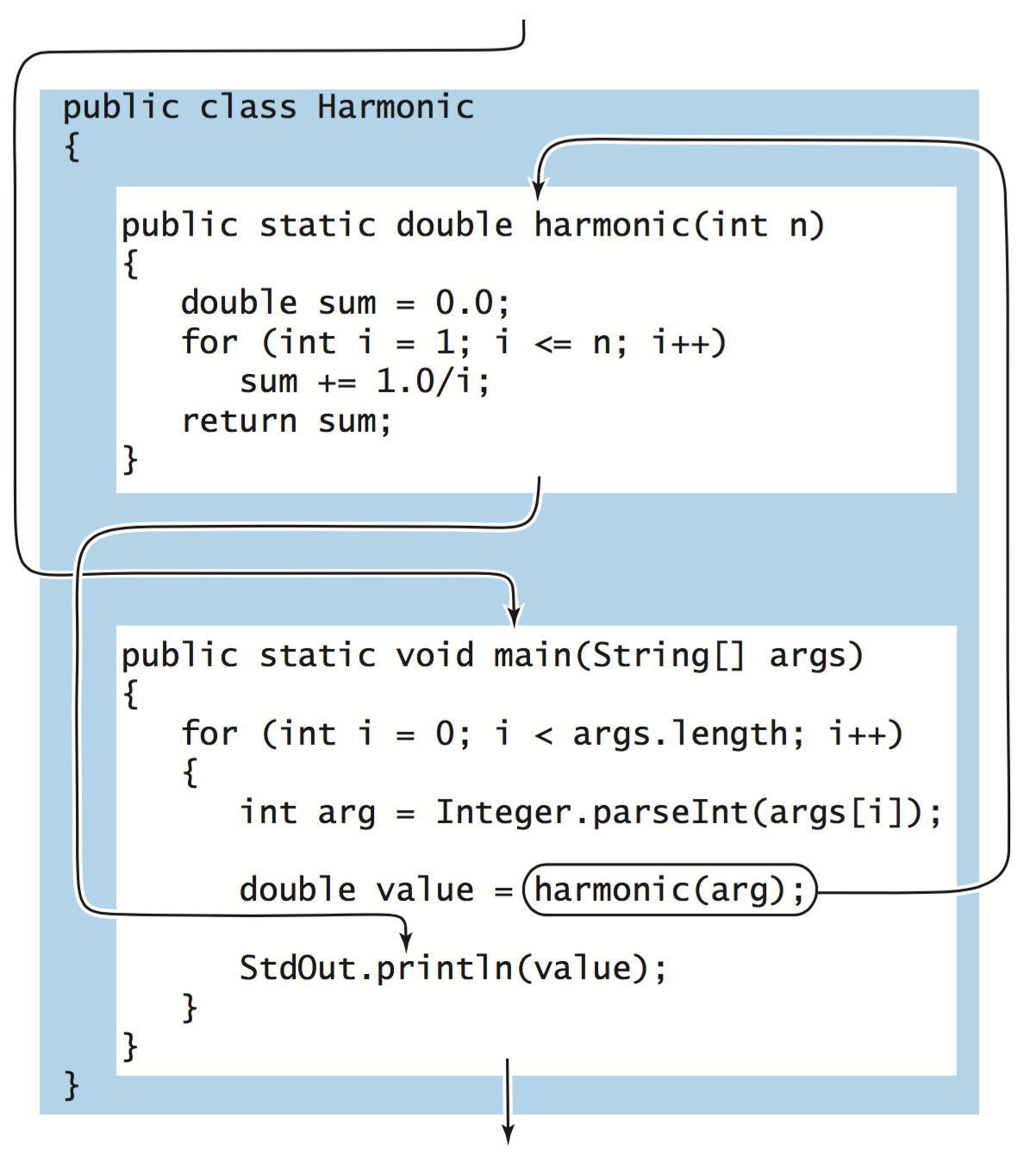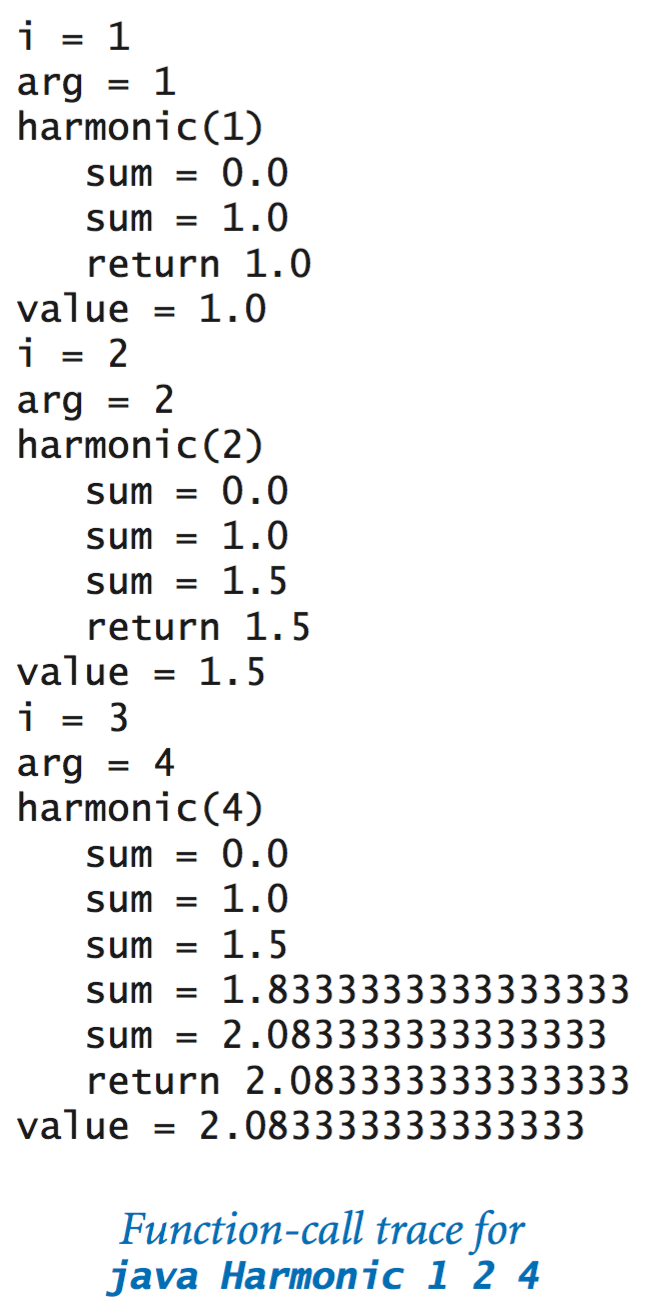• Function-call trace. One simple approach to following the flow of control through function calls is to imagine that each function prints its name and argument value when it is called and its return value just before returning, with indentation added on calls and subtracted on returns.
• Static method definition. The first line of a static method definition, known as the signature, gives a name to the method and to each parameter variable. It also specifies the type of each parameter variable and the return type of the method. Following the signature is the body of the method, enclosed in curly braces. The body consists of the kinds of statements we discussed in Chapter 1. It also can contain a return statement, which transfers control back to the point where the static method was called and returns the result of the computation or return value. The body may declare local variables, which are variables that are available only inside the method in which they are declared.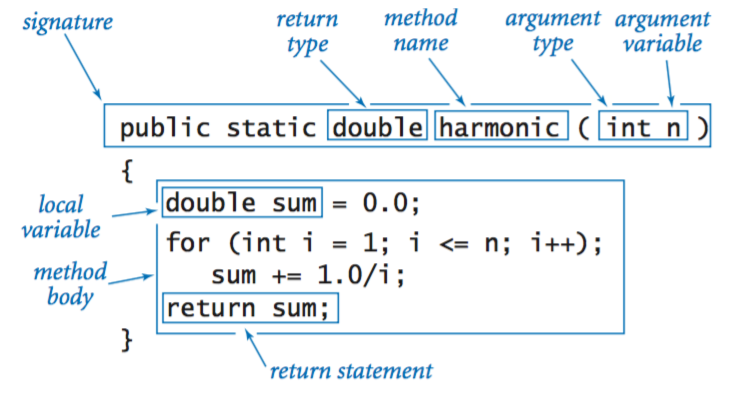• Function calls. A static method call is nothing more than the method name followed by its arguments, separated by commas and enclosed in parentheses. A method call is an expression, so you can use it to build up more complicated expressions. Similarly, an argument is an expression—Java evaluates the expression and passes the resulting value to the method. So, you can write code like Math.exp(-x*x/2) / Math.sqrt(2*Math.PI) and Java knows what you mean.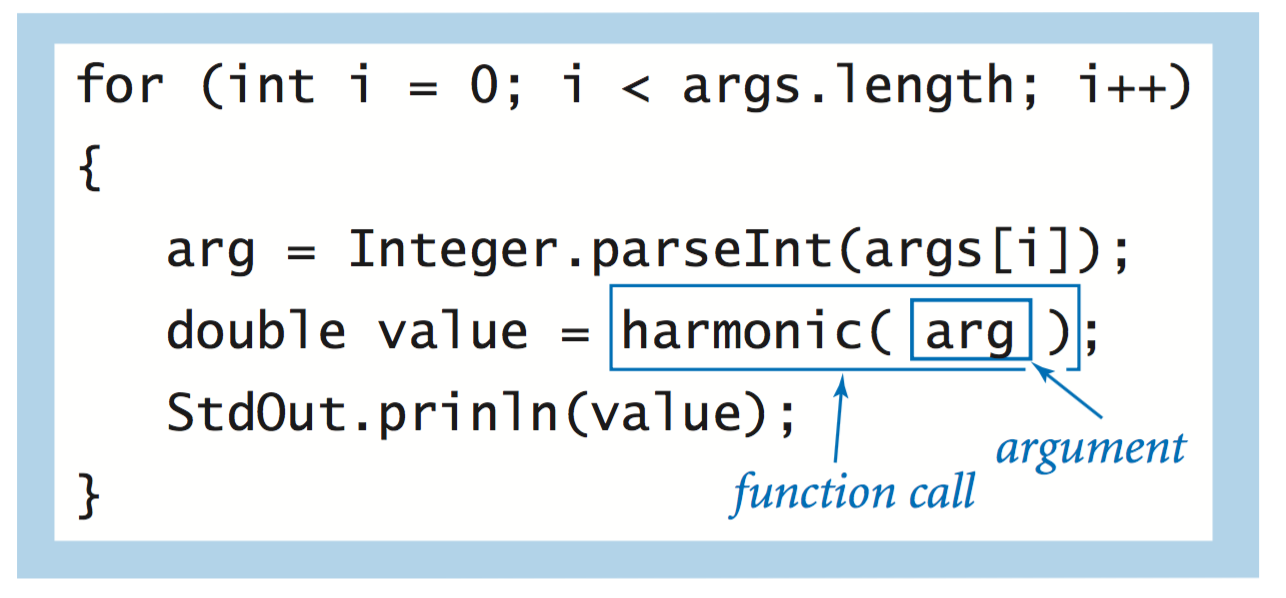## Properties of static methods.

• Multiple arguments. Like a mathematical function, a Java static method can take on more than one argument, and therefore can have more than one parameter variable.

• Multiple methods. You can define as many static methods as you want in a .java file. These methods are independent and can appear in any order in the file. A static method can call any other static method in the same file or any static method in a Java library such as Math.

• Overloading. Static methods whose signatures differ are different static methods. Using the same name for two static methods whose signatures differ is known as overloading.

• Multiple return statements. You can put return statements in a method wherever you need them: control goes back to the calling program as soon as the first return statement is reached.

• Single return value. A Java method provides only one return value to the caller, of the type declared in the method signature.

• Scope. The scope of a variable is the part of the program that can refer to that variable by name. The general rule in Java is that the scope of the variables declared in a block of statements is limited to the statements in that block. In particular, the scope of a variable declared in a static method is limited to that method's body. Therefore, you cannot refer to a variable in one static method that is declared in another.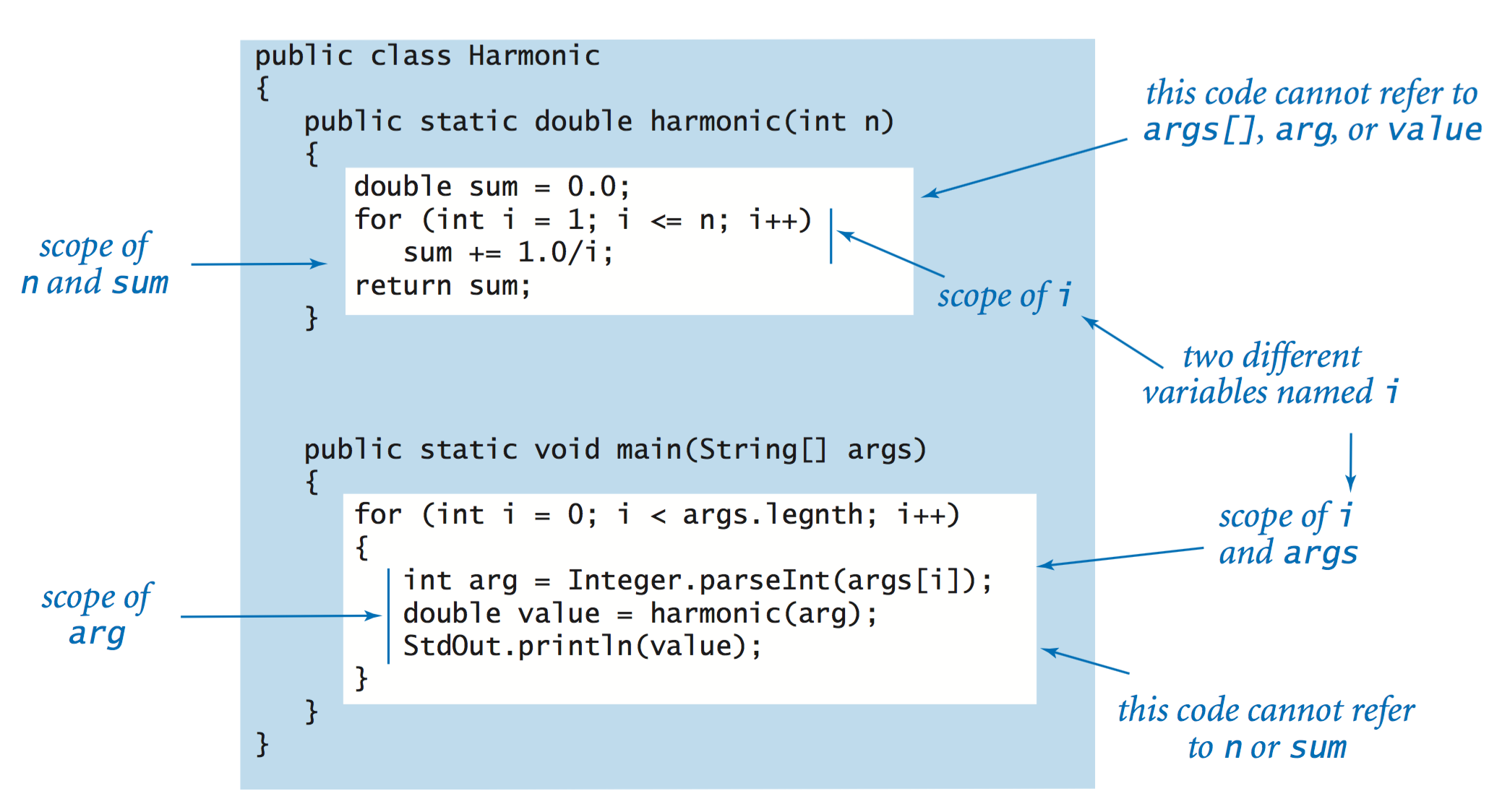• Side effects. A pure function is a function that, given the same arguments, always returns the same value, without producing any observable side effects, such as consuming input, producing output, or otherwise changing the state of the system. The function harmonic() is an example of a pure function. However, in computer programming, we often define functions whose only purpose is to produce side effects. In Java, a static method may use the keyword void as its return type, to indicate that it has no return value.
FunctionExamples.java gives a number of examples.

## Implementing mathematical functions.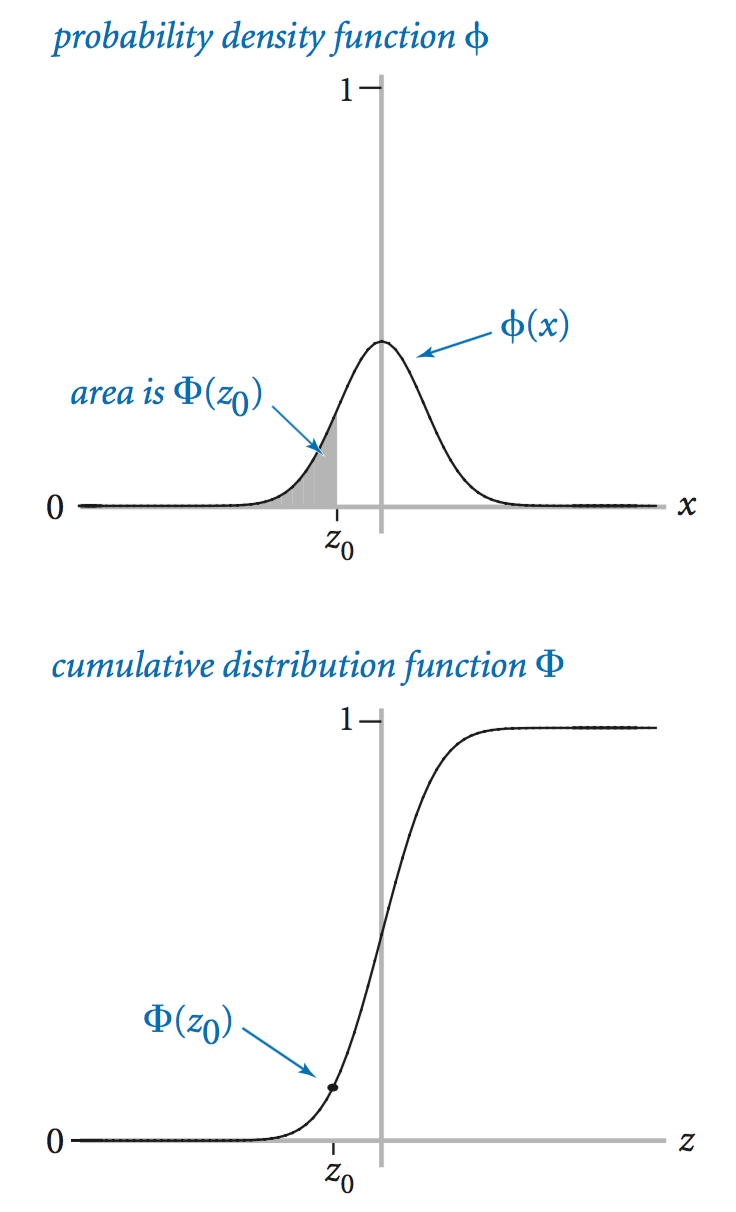We now consider two important functions that play an important role in science, engineering, and finance. The Gaussian (normal) distribution function is characterized by the familiar bell-shaped curve and defined by the formula:
$$\phi(x) \;=\; \frac{1}{\sqrt{2 \pi}} e^{-x^2/2}$$
and the cumulative Gaussian distribution function $$\Phi(z)$$ is defined to be the area under the curve defined by $$\phi(x)$$ above the x-axis and to the left of the vertical line x = z.
• Closed form. In the simplest situation, we have a closed-form mathematical equation defining our function in terms of functions that are implemented in the Math library. This is the case for $$\phi(x)$$.
• No closed form. Otherwise, we may need a more complicated algorithm to compute function values. This situation is the case for $$\Phi(z)$$, for which no closed-form expression exists. For small (respectively large) z, the value is extremely close to 0 (respectively 1); so the code directly returns 0 (respectively 1); otherwise the following Taylor series approximation is an effective basis for evaluating the function:
$$\begin{eqnarray*} \Phi(z) &= & \int_{-\infty}^{z} \phi(x) dx \\ & = & \frac{1}{2} \;+\; \phi(z) \; \left(z \;+\; \frac{z^3}{3} \;+\; \frac{z^5}{3 \cdot 5} \;+\; \frac{z^7}{3 \cdot 5 \cdot 7} \;+\; \ldots \;\; \right) \end{eqnarray*}$$
Gaussian.java implements both of these static methods.

## Using static methods to organize code.

With the ability to define functions, we can better organize our programs by defining functions within them when appropriate. For example, Coupon.java is a version of CouponCollector.java that better separates the individual components of the computation.
• Given n, compute a random coupon value.
• Given n, do the coupon collection experiment.
• Get n from the command line, and then compute and print the result.
Whenever you can clearly separate tasks in programs, you should do so.

## Passing arguments and returning values.

Next, we examine the specifics of Java's mechanisms for passing arguments to and returning values from functions.
• Pass by value. You can use parameter variables anywhere in the code in the body of the function in the same way you use local variables. The only difference between a parameter variable and a local variable is that Java evaluates the argument provided by the calling code and initializes the parameter variable with the resulting value. This approach is known as pass by value.
• Arrays as arguments. When a static method takes an array as an argument, it implements a function that operates on an arbitrary number of values of the same type.
• Side effects with arrays. It is often the case that the purpose of a static method that takes an array as argument is to produce a side effect (change values of array elements).
• Arrays as return values. A static method can also provide an array as a return value.
ArrayFunctionExamples.java gives a number of examples of arrays as arguments to and return values from functions.

## Superposition of sound waves.

Notes like concert A have a pure sound that is not very musical, because the sounds that you are accustomed to hearing have many other components. Most musical instruments produce harmonics (the same note in different octaves and not as loud), or you might play chords (multiple notes at the same time). To combine multiple sounds, we use superposition: simply add the waves together and rescale to make sure that all values stay between −1 and 1.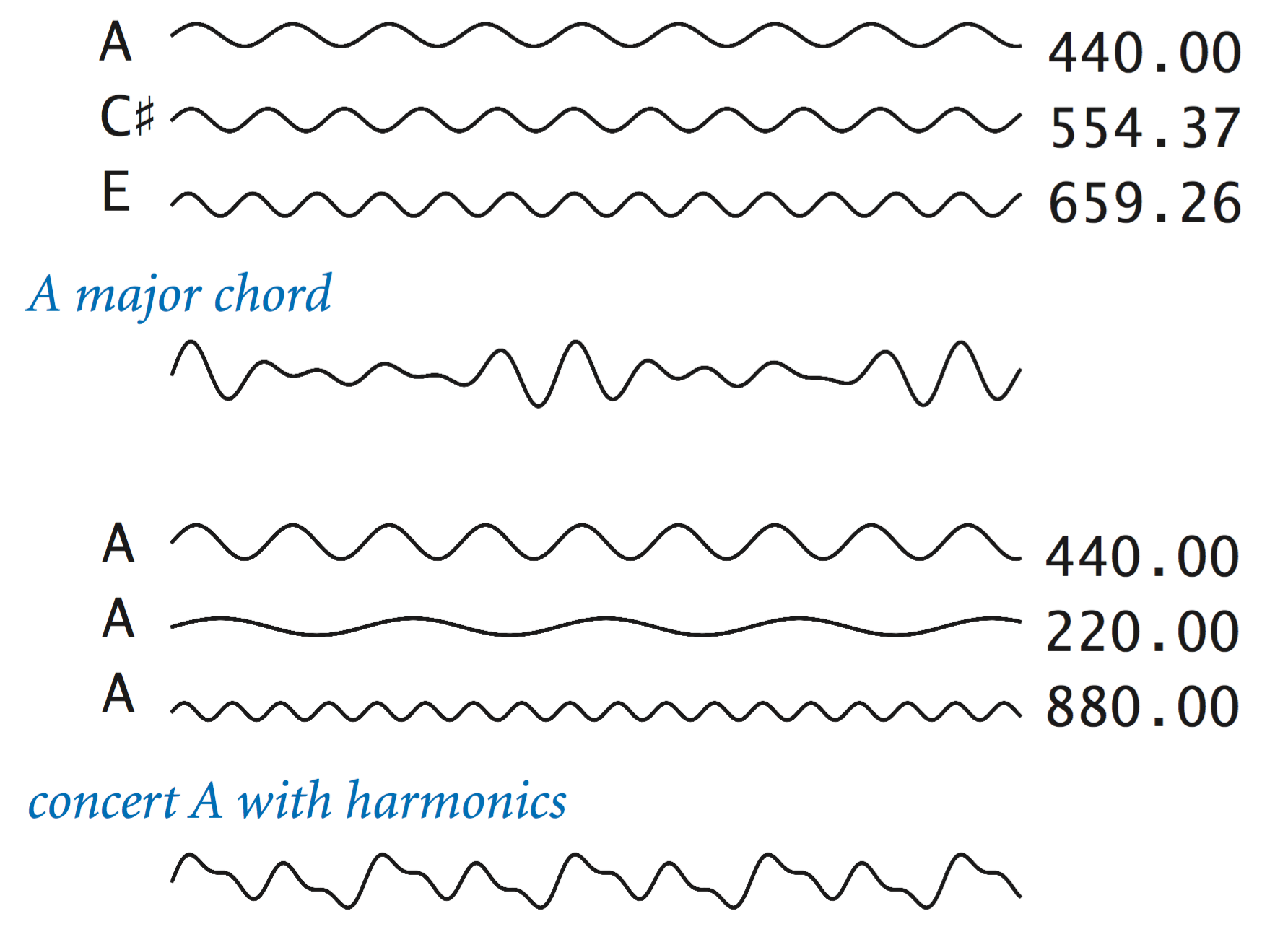PlayThatTuneDeluxe.java is a version of PlayThatTune that encapsulates the sound wave calculation and adds harmonics.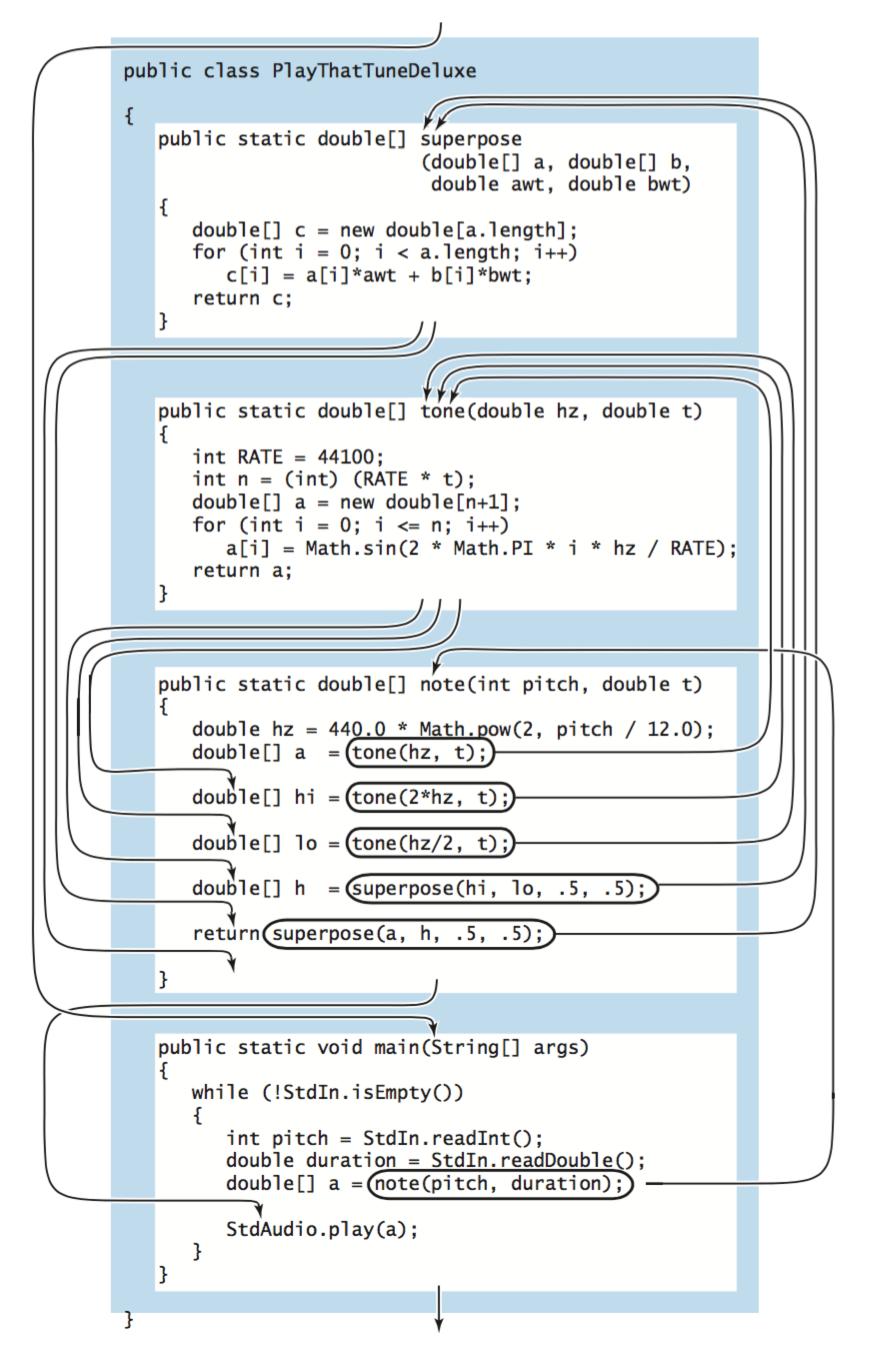Here a few sample data files (created by various students):

#### Exercises

1. Write a static method max3() that takes three int arguments and returns the value of the largest one. Add an overloaded function that does the same thing with three double values.

Solution:

 public static int max3(int a, int b, int c) { int max = a; if (b > max) max = b; if (c > max) max = c; return max; } public static double max3(double a, double b, double c) { double max = a; if (b > max) max = b; if (c > max) max = c; return max; } 
2. Write a static method majority() that takes three boolean arguments and returns true if at least two of the arguments are true, and false otherwise. Do not use an if statement.

Solution:

 public static boolean majority(boolean a, boolean b, boolean c) { return (a && b) || (a && c) || (b && c); } 
3. Write a static method eq() that takes two int arrays as arguments and returns true if the arrays have the same length and all corresponding pairs of elements are equal, and false otherwise.

Solution. ArraysEquals.java.

4. Write a static method sqrt() that takes a double argument and returns the square root of that number. Use Newton's method (see Sqrt.java) to compute the result.

Solution: Newton.java.

5. Consider the static method cube() below.
 public static void cube(int i) { i = i * i * i; } 
How many times is the following for loop iterated?
 for (int i = 0; i < 1000; i++) cube(i); 

#### Creative Exercises

1. Black–Scholes option valuation. The Black–Scholes formula supplies the theoretical value of a European call option on a stock that pays no dividends, given the current stock price s, the exercise price x, the continuously compounded risk-free interest rate r, the volatility σ, and the time (in years) to maturity t. The value is given by the formula

$$\Phi(a) - s x e^{-rt} \, \Phi(b)$$

where

$$a = \frac{\ln(s/x) + (r + \sigma^2 / 2) t}{\sigma \sqrt{t}}, \;\; b = a - \sigma \sqrt{t}$$

Write a program BlackScholes.java that takes s, x, r, sigma, and t from the command line and prints the Black-Scholes value.

2. Calendar. Write a program Calendar.java that takes two integer command-line arguments m and y and prints the monthly calendar for the month m and year y, as in this example:
  February 2009 S M Tu W Th F S 1 2 3 4 5 6 7 8 9 10 11 12 13 14 15 16 17 18 19 20 21 22 23 24 25 26 27 28 

Hint: See LeapYear.java and Exercise 1.2.29.

3. Horner's method. Write a class Horner.java with a method evaluate() that takes a floating-point number xp[] as arguments and returns the result of evaluating the polynomial whose coefficients are the elements in p[] at x:
$$p_0 + p_1x^1 + p_2x^2 + \; \ldots \; + p_{n-2}x^{n-2} + p_{n-1}x^{n-1}$$
Use Horner's method, an efficient way to perform the computations that is suggested by the following parenthesization:
$$p_0 + x (p_1 + x (p_2 + \; \ldots \; + x(p_{n-2} + x p_{n-1}) \ldots))$$
Write a test client with a static method exp() that uses evaluate() to compute an approximation to e^x, using the first n terms of the Taylor series expansion $$e^x = 1 + x + x^2/2! + x^3/3! + \ldots$$ Your client should take a command-line argument x and compare your result against that computed by Math.exp().
4. Benford's law. The American astronomer Simon Newcomb observed a quirk in a book that compiled logarithm tables: the beginning pages were much grubbier than the ending pages. He suspected that scientists performed more computations with numbers starting with 1 than with 8 or 9, and postulated the first digit law, which says that under general circumstances, the leading digit is much more likely to be 1 (roughly 30%) than the digit 9 (less than 4%). This phenomenon is known as Benford's law and is now often used as a statistical test. For example, IRS forensic accountants rely on it to discover tax fraud. Write a program Benford.java that reads in a sequence of integers from standard input and tabulates the number of times each of the digits 1–9 is the leading digit, breaking the computation into a set of appropriate static methods. Use your program to test the law on some tables of information from your computer or from the web. Then, write a program to foil the IRS by generating random amounts from $1.00 to$1,000.00 with the same distribution that you observed.

#### Web Exercises

1. Diamond tile. Write a program DiamondTile.java that takes a command-line argument N and creates an N-by-N tile of diamonds. Include static methods diamond() and filledDiamond().
2. Hexagonal tile. Write a program HexTile.java that takes a command-line argument N and creates an N-by-N tile of hexagons. Include static methods hexagon() and filledHexagon().
3. Inverse Gaussian cumulative distribution. Suppose SAT math scores are normally distributed with mean 500 and standard deviation. Estimate how high student must score in order to be among the top 10%. To do this you need to find the value z for which Φ(z, 500, 100) = 0.9. Hint: use binary search.
4. SAT scores. A prominent northeastern university receives 20,000 student applications. Assume that the SAT scores of these individuals is normally distributed with mean 1200 and standard deviation 100. Suppose the university decides to admit the 5,000 students with the best SAT scores. Estimate the lowest score that will still be admitted.
5. Voting machines. Suppose that in a population of 100 million voters, 51% vote for candidate A and 49% vote for candidate B. However, the voting machines are prone to make mistakes, and 5% of the time they produce the wrong answer. Assuming the errors are made independently and at random, is a 5% error rate enough to invalidate the results of a close election? What error rate can be tolerated?
6. Gambler's histogram. Write a program RandomWalk.java that takes one command line parameter M and simulates a gambler starting with M who places exactly M one dollar bets.
1. Produce a histogram of the amount of money the gambler ends up with by running this experiment N times.
2. The amount of money the gambler ends up with follows a binomial distribution with mean M and variance N/4. The distribution can be approximated by a normal distribution with the same mean and variance. Produce a histogram for the fraction of time you'd expect the gambler to end up with the amount in each histogram bin.
Organize your program up into several functions.
7. Statistical sampling. Write a program Sampling.java takes a random sample of N people and asks them a yes/no question. Compute a 95% confidence interval.
8. Blackjack. Write a program Blackjack.java that plays the basic strategy or write a program BlackjackCounter.java that implements the high-low card counting system.
9. Wavelets. Applications to computer vision, human vision, speech processing, compressing the FBI fingerprint database, filtering noisy data, detecting self-similarity in time series, sound synthesis, computer graphics, medical imaging, analyzing the clumping of galaxies, and analyzing turbulence. The Haar function is definite by Φ(x) = 1 if 0 ≤ x < 1/2, Φ(x) = -1 if 1/2 ≤ x < 1, and Φ(x) = 0 otherwise. For integer m and n, the Haar basis function Φm,n(x) = 2-m/2 Φ(2-mx - n). Write a program Haar.java that takes two integer input M and N, and one real input x and prints Φm,n(x). Or maybe plot it?
10. Baccarat. Baccarat is a simple card game which has been romanticized in James Bond movies. When the player is dealt nine, the croupier proclaims "neuf a la banque". Write a program that determines your chance of winning....
11. Collinear points. Write a function
 public boolean areCollinear(int x1, int y1, int x2, int y2, int x3, int y3) 

that returns true if the three points (x1, y1), (x2, y2), and (x3, y3) lie on the same line, and false otherwise.

12. Gauss error function. The error function is a function that arises in probability, statistics, and solutions to differential equations. For example, Φ(z) = 1/2 + (1 + erf(z / sqrt(2))), where Φ(z) is the Gaussian cumulative distribution function defined above.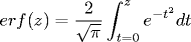The integral has no closed form solution in terms of elementary functions so we resort to approximations. When z is nonnegative, the Chebyshev fitting estimate below is accurate to 7 significant digits: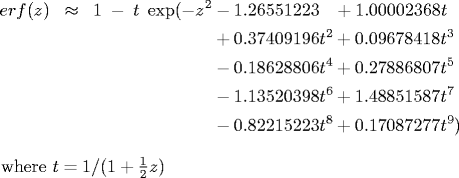If z is negative, use the identity erf(z) = -erf(-z). A particularly efficient way to implement it is via a judicious use of parentheses known as Horner's method. Write a function erf() in ErrorFunction.java takes one real input z and computes the error function using the formula above.

13. Haversine. Write a function haversine() that takes one double argument θ and returns haversine(θ) = (sin(θ/2))^2.
14. Soil temperature. (Cleve Moler) Suppose the soil temperature is uniformly Ti (20 degrees C) at the surface and beneath. A cold front moves in and the surface temperature Ts (-15 C) remains for the foreseeable future. The temperature T(x, t) at time t seconds of the soil at x meters beneath the surface is given by T(x, t) = Ts + (Ti - Ts) erf(x / 2 sqrt(alpha t)), where alpha (0.138 * 10^-6 m^2 / s) is the thermal conductivity of the soil. What is the temperature at 10 meters after 30 days of exposure to these conditions? How long until water freezes (0 degrees) at 5 meters beneath the surface? How deep to dig a water main so that it can withstand 60 days of exposure to these conditions without freezing?
15. Craps. Calculate the probability of winning a pass bet in craps. Here are the rule for a pass bet. Roll two 6-sided dice, and let x be their sum.
• if x is 7 or 11, you win instantly
• if x is 2, 3, or 12, you lose instantly
• otherwise, repeatedly roll two dice until their sum is either x or 7
• if their sum is x, you win
• if their sum is 7, you lose

Program Craps.java takes a command line parameter N and simulates N pass bets. The program's organization benefits from two helper functions: sumOfTwoDice and winsPassBet. Both functions have one interesting feature - they do not take any input arguments. The first function simulate the throw of two dice. This returns an integer between 2 and 12, but not all values are equally likely. To simulate the probabilities correctly, we call StdRandom.random(6) twice and one to generate a number between 1 and 6. Then, we add the two values. The second function returns a boolean value: true if we win the pass bet and false otherwise. This function has several return statements. As soon as the first one is executed, the function terminates with the given return value.

16. Musical scale. Using the function note(), write a program Scale.java to play a major scale.
17. Primality testing. Create a function isPrime() that takes an integer argument N and returns true or false depending on whether N is prime.
 public static boolean isPrime(long N) { if (N < 2) return false; for (long i = 2; i*i <= N; i++) { if (N % i == 0) return false; } return true; } 
18. Electronic funds transfer routing number check. Given a 9 digit EFT routing number a1a2a3a4a5a6a7a8a9 the check equation is
3 a1 + 7a2 + a3 + 3a4 + 7a5 + a6 +3a7 +7a8 +a9 mod 10 = 0
Check digit reference.
19. Write a static method nint() that takes a real number as a parameter and returns the nearest integer. Do not use any Math library function, instead use casting.
20. Write a static method int mod(int a, int n), where a is an integer and n is a positive integer, and returns a mod n. This corresponds to a % n when a is positive, but if a is negative, a % n returns a non-positive integer.
21. Present value.
1. Write a method fv that computes the amount of money you will have if you invest C dollars today at the compound interest rate of r per period, in T periods. The formula for the future value is given by C*(1 + r)^T.
2. Write a method pv that computes the amount of money that would have to be invested now, at the compound interest rate r per period, to obtain a cash flow of C in T periods. The formula for the present value is given by C/(1 + r)^T.
22. The ACT is another standardized test. Assume that test scores follow a Gaussian distribution with mean 18 and standard deviation 6. Also assume that the test takers for SAT and ACT are indistinguishable. Which is better, an SAT score of 620 or ACT of 26?
23. Write a program Factorial.java that takes one integer command line input n and prints out n! = 1 * 2 * ... * n. Write a function that has the following signature:
 public static long factorial(int n) 

What is the largest value of n that your function can handle without overflow?

24. What is wrong with the following function?
 static int sum(int n) { if (n < 0) return; double sum = 0.0; for (int i = 0; i < n; i++) sum = sum + i; return sum; } 

Answer: The function is declared to return a value of type int. The first return statement is wrong since it returns nothing. The second return statement is wrong since it returns a value of type double.

25. What does the following do?
 public static void negate(int a) { a = -a; } public static int main(String[] args) { int a = 17; System.out.println(a); negate(a); System.out.println(a); } 

Answer: It prints 17 twice. A function cannot change the value of a primitive type variable in another function.

26. Write a function that takes three real arguments, x, y, and s, and plots an equilateral triangle centered on (x, y) with side length s. Call the function a number of times in main to produce an entertaining pattern.
27. Which of the following functions returns the minimum of its four inputs? Which is easiest to understand and verify that it is correct?
 public static int min(int a, int b, int c, int d) { // if a is the smallest return it if (a <= b && a <= c && a <= d) return a; // otherwise, if b is the smallest of b, c, and d, return it if (b <= c && b <= d) return b; // otherwise, return the smaller of c and d if (c <= d) return c; return d; } public static int min(int a, int b, int c, int d) { int min = a; if (b < min) min = b; if (c < min) min = c; if (d < min) min = d; return min; } public static int min(int a, int b, int c, int d) { if (a < b && a < c && a < d) return a; if (b < c && b < d) return b; if (c < d) return c; return d; } public static int min(int a, int b, int c, int d) { if (a <= b) { if (a <= c) { if (a <= d) return a; else return d; } if (c <= d) return c; else return d; } if (b <= c) { if (b <= d) return b; else return d; } else if (c <= d) return c; return d; } public static int min(int a, int b) { if (a <= b) return a; else return b; } public static int min(int a, int b, int c, int d) { return min(min(a, b), min(c, d)); } 

28. How would you go about testing whether one of the functions in the previous exercise does what it claims to do?

Answer: you can't hope to test it on every conceivable input since there are 2128 different possible inputs. Instead, test it on all 4! = 24 cases depending on whether a < b, a < c, ..., c < d. Or all 4^4 permutations of 0, 1, 2, and 3.

29. What's wrong with the following method call?
 double y = 2.0; double x = sqrt(double y); 

It re-declare the variable y when calling sqrt().

30. Sawtooth. Write a program SawTooth.java to plot 2/pi [sin(1t)/1 + sint(2t)/2 + sin(3t)/3 + ... ]. As you plot more and more terms, the wave converges to a sawtooth wave. Then play it using standard audio.
31. Square wave. Plot 4/pi [sin(1*2*pi*t)/1 + sint(3*2*pi*t)/3 + sin(5*2*pi*t)/5 + ... ]. As you plot more and more terms, the wave converges to a square wave. Then play it using standard audio.
32. Write a program to print the lyrics to Old McDonald.
 public static String sing(String animals, String sound) { String s = ""; s += "Old MacDonald had a farm\n"; s += "E-I-E-I-O\n"; s += "And on his farm he had some " + animals + "\n"; s += "With a " + sound + "-" + sound + " here\n"; s += "And a " + sound + "-" + sound + " there\n"; s += "Here a " + sound + ", there a " + sound + "\n"; s += "Everywhere a + sound + "-" + sound + "\n"; s += "Old MacDonald had a farm\n"; s += "E-I-E-I-O\n"; return s; } ... System.out.println(sing("chicks", "cluck")); System.out.println(sing("cows", "moo")); System.out.println(sing("pigs", "oink")); System.out.println(sing("cats", "meow")); System.out.println(sing("sheep", "baa")); System.out.println(sing("ducks", "quack")); System.out.println(sing("horses", "neigh")); 
33. Write a static method maxwellBoltzmann() that returns a pseudo-random value from a Maxwell-Boltzmann distribution with parameter σ. To produce such a value, take the sum of the squares of three Gaussian random variables with mean 0 and standard deviation σ, and return the square root. The speeds of molecules in an ideal gas have a Maxwell-Boltzmann distribution, where the parameter σ is related to XYZ.
34. Write a static method reverse1() that takes a string array as an argument and creates a new array with the entries in reverse order (and does not change the order of the entries in the argument array). Write a static method reverse2() that takes a string array as an argument and reverses its entries. Put your code in a program Reverse.java.
35. Which function gets called by f(1, 2) if I have two overloaded functions
 public static void f(int x, double y) { System.out.println("f(int, double)"); } public static void f(double x, int y) { System.out.println("f(double, int)"); } 

Solution. Ordinarily, Java's type promotion rules would promote either int argument to double. However, in this case, that would result in two matching overloaded signatures. Since Java can't resolve the ambiguity, Overloaded.java results in a compile-time error.

36. Gaussian random values. Experiment with the following method for generating random variables from the Gaussian distribution, which is based on generating a random point in the unit circle and using a form of the Box-Muller transform. (see Exercise 1.2.27 and the discussion of do-while at the end of Section 1.3).
 public static double gaussian() { double r, x, y; do { x = uniform(-1.0, 1.0); y = uniform(-1.0, 1.0); r = x*x + y*y; } while (r >= 1 || r == 0); return x * Math.sqrt(-2.0 * Math.log(r) / r); } 

Take a command-line argument N and generate N random numbers, using an array a to count the numbers generated that fall between i*.05 and (i+1)*.05 for i from 0 to 19. Then use StdDraw to plot the values and to compare your result with the normal bell curve.

Remark: This method is preferred over the one described in Exercise XYZ for both efficiency and accuracy. Although it involves a loop, the do-while loop is executed only 4 / π = 1.273 times on average. This reduces the overall expected number of calls to transcendental functions.